Browse by Topic
Related Topics

# The Singular Euler–Maclaurin Expansion A New Twist to a Centuries-Old Problem## On Sums and Integrals

Of all mathematical operations, addition is the most basic: It’s what we learn first in school. Historically, it is the most ancient. While the simple task of getting the sum of two numbers is simple, sums of many numbers can easily turn into a challenging numerical problem if the number of summands is very large.

Large sums appear frequently in nature. Consider, for instance, a solid body, which consists of a number of atoms of the order of magnitude of the Avogadro number N ≈ 1023. If we want to compute long-range forces, e.g. due to the Coulomb interaction between charged particles, we will have to evaluate a sum over 1023 summands, which is numerically infeasible.

How can we compute large (or even infinite) sums efficiently? A first attempt is to approximate a sum by an integral: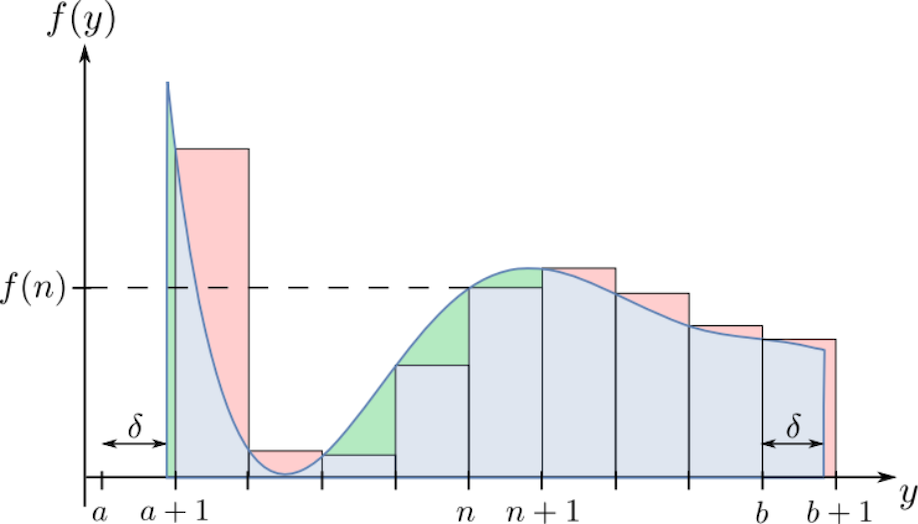The sum over the values of the function f (rectangles) is approximated by an integral (blue curve). But we see that in some parts of the geometry, the integral overestimates (green parts) or underestimates (red parts) the sum. But how precise is this integral approximation? Is it reliable? Can we improve upon it?

A first attempt at giving an answer to this fundamental mathematical question was given almost three hundred years ago by the famous mathematicians Leonhard Euler and Colin Maclaurin. They independently discovered the Euler–Maclaurin summation formula that relates sums to associated integrals, a highly important result that is used in numerical practice to this day, and is implemented as the method EulerMaclaurin in Mathematica’s NSum routine. The formula reads: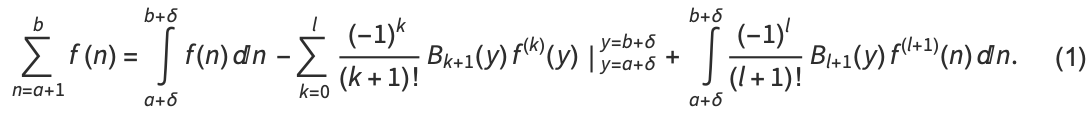The sum is approximated by an integral with an arbitrary offset δ ∈ (0, 1]. Higher-order corrections to the integral approximation are described by derivatives of the summand function at the boundaries of integration. The coefficients of these derivatives are formed by the periodic Bernoulli functions Bk.

The Bernoulli polynomials, which coincide with the periodic Bernoulli functions on (0, 1], are implemented in Mathematica as BernoulliB. We periodically extend these functions to the real line, noting that the l = 1 functions are discontinuous at the integers (the argument 1+y–Ceiling[y] is chosen such that the left-sided limit always exists):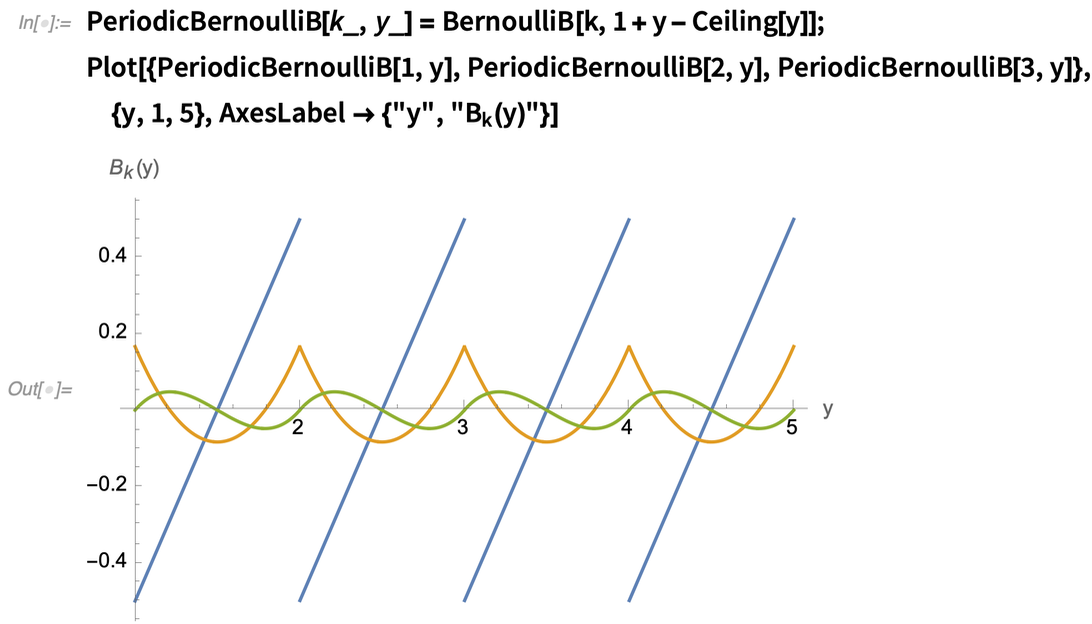✕ ```PeriodicBernoulliB[k_, y_] = BernoulliB[k, 1 + y - Ceiling[y]]; Plot[{PeriodicBernoulliB[1, y], PeriodicBernoulliB[2, y], PeriodicBernoulliB[3, y]}, {y, 1, 5}, AxesLabel -> {"y", "\!\(\*SubscriptBox[\(B\), \(k\)]\)(y)"}]```

The convergence of the summation formula is determined by the asymptotic behavior of the Bernoulli periodic functions Bk as k → ∞. We can quickly analyze this behavior by creating a table of the maximum absolute value of the periodic Bernoulli functions: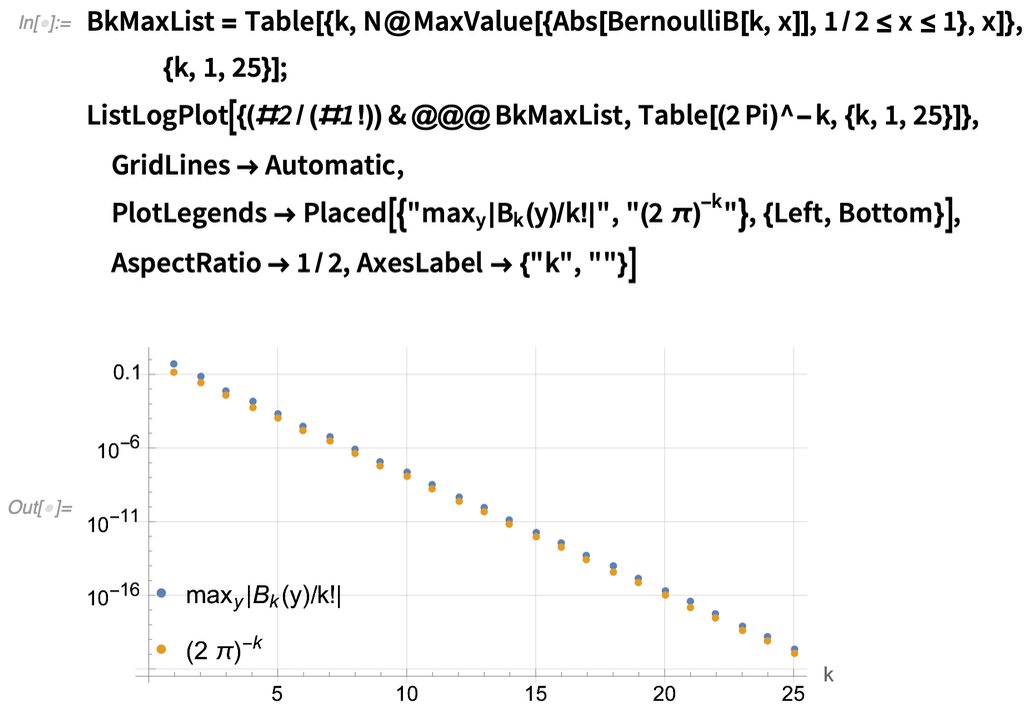✕ ```BkMaxList = Table[{k, N@MaxValue[{Abs[BernoulliB[k, x]], 1/2 <= x <= 1}, x]}, {k, 1, 25}]; ListLogPlot[{(#2/(#1!)) & @@@ BkMaxList, Table[(2 Pi)^-k, {k, 1, 25}]}, GridLines -> Automatic, PlotLegends -> Placed[{"\!\(\*SubscriptBox[\(max\), \ \(y\)]\)|\!\(\*SubscriptBox[\(B\), \(k\)]\)(y)/k!|", "(2Ê\[Pi]\!\(\*SuperscriptBox[\()\), \(-k\)]\)"}, {Left, Bottom}], AspectRatio -> 1/2, AxesLabel -> {"k", ""}]```

The previous graph shows the well-known estimatewhich implies the important condition that the Euler–Maclaurin expansion converges if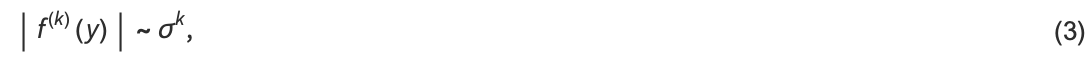with σ < 2 π. This corresponds to band-limited functions f with sufficiently small bandwidth in Fourier space. If the function f includes an algebraic singularity, e.g. f(y) = | y |–v, however, the derivatives scale as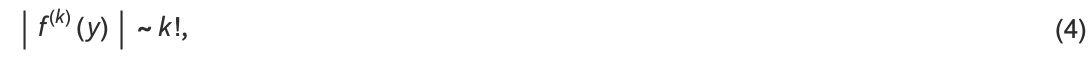and the remainder of the expansion diverges for k → ∞.

We can verify the asymptotic scaling of the singular interaction using Mathematica’s Asymptotic function: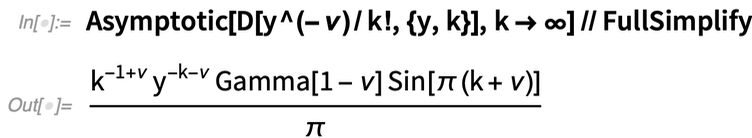✕ `Asymptotic[D[y^(-\[Nu])/k!, {y, k}], k -> \[Infinity]] // FullSimplify`

The expansion is thus only of limited use if the summand function includes singularities. You might rightfully ask, “Why should we care about singular functions? Are such functions of high enough practical relevance that we should worry about them?” It turns out that they are.

## A Short Story of the Fundamental Forces

As far as we know, there are four fundamental forces in physics. Among those four, two are long ranged, namely the electromagnetic and gravitational interactions. (We will not consider the weak and strong interactions for now, as they act only on nuclear scales.) Not only do these forces decay slowly with distance, they are also singular. Both the gravitational interaction and the electrostatic energy V of two particles separated by a distance-y scale asand the respective forces F follow an inverse square law: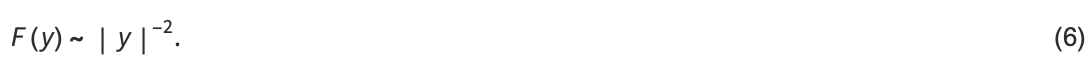Thus, if two particles come infinitely close, both the potential energy and the resulting forces diverge. From these fundamental interactions, more general interactions can arise, e.g.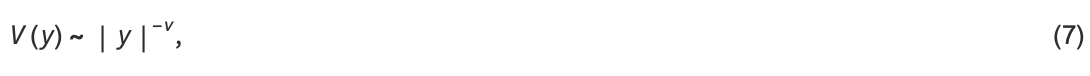where for instance v = 3 describes the dipole interaction between magnetic particles.

In nature, we rarely deal with small numbers of particles (atoms or molecules). In most cases, e.g. in condensed matter systems, the system at hand is formed by a very, very large number of particles. The mutual interactions between the particles create the properties of the solid as a whole. If we want to compute the forces in a solid, however, we have to evaluate sums of the kind (e.g. in 1D)where u describes the displacement of a particle from a crystal with equal nearest-neighbor distances. If the number of particles N is large, computing this sum is a very hard task, even if the displacement function u is known. It is now natural to try to approximate this complicated force sum by an integral and to write the finite-size corrections by means of the Euler–Maclaurin expansion. Yet unfortunately, F is singular, and thus the error term in the Euler–Maclaurin summation diverges. This in the end leads to an uncontrolled error and results, in general, in a bad approximation. The three-hundred-year-old reliable workhorse of the numerical analysis of large sums, the Euler–Maclaurin expansion, reaches its limits when trying to tackle the sums that appear in modern physics. What we need is a new expansion, one that passes the test where the old expansion fails.

## The Singular Euler–Maclaurin Expansion

We now improve the original Euler–Maclaurin expansion and make it applicable to singular functions that appear in condensed matter physics. But how can the seemingly unavoidable divergence of the expansion be remedied? We consider functions f of the form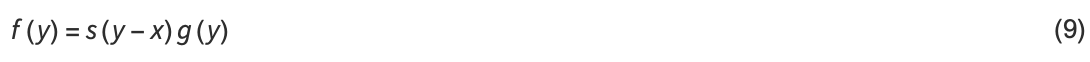where s(y) = | y |–v and x = ∈ ℤ. We call s the interaction and g the interpolating function. As we have seen before, the divergence of the remainder term arises due to taking derivatives of the interaction. Our strategy in the following is to avoid these derivatives and to take derivatives of the function g instead. The interaction is included in the coefficients of these derivatives. These coefficients are formed by a generalization of the periodic Bernoulli functions, which we call Bernoulli-A functions.

We implement the Bernoulli-A functions as given in eq. (12) of ref.  using Mathematica’s efficient implementation of the Hurwitz zeta function: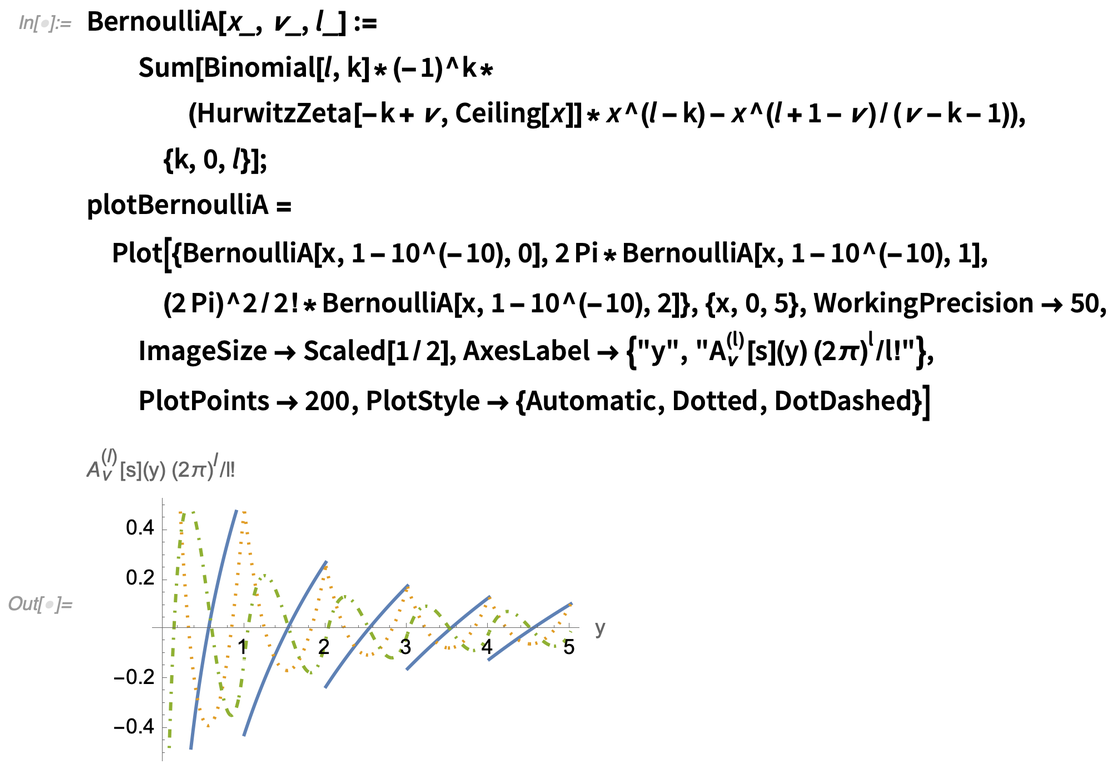✕ ```BernoulliA[x_, \[Nu]_, l_] := Sum[Binomial[l, k]*(-1)^ k*(HurwitzZeta[-k + \[Nu], Ceiling[x]]*x^(l - k) - x^(l + 1 - \[Nu])/(\[Nu] - k - 1)), {k, 0, l}]; plotBernoulliA = Plot[{BernoulliA[x, 1 - 10^(-10), 0], 2 Pi*BernoulliA[x, 1 - 10^(-10), 1], (2 Pi)^2/2!* BernoulliA[x, 1 - 10^(-10), 2]}, {x, 0, 5}, WorkingPrecision -> 50, ImageSize -> Scaled[1/2], AxesLabel -> {"y", "\!\(\*SubsuperscriptBox[\(A\), \(\[Nu]\), \((l)\)]\)[s](y) (2\ \[Pi]\!\(\*SuperscriptBox[\()\), \(l\)]\)/l!"}, PlotPoints -> 200, PlotStyle -> {Automatic, Dotted, DotDashed}]```

The Bernoulli-A functions remain well defined in the limit vz for z ∈ ℤ: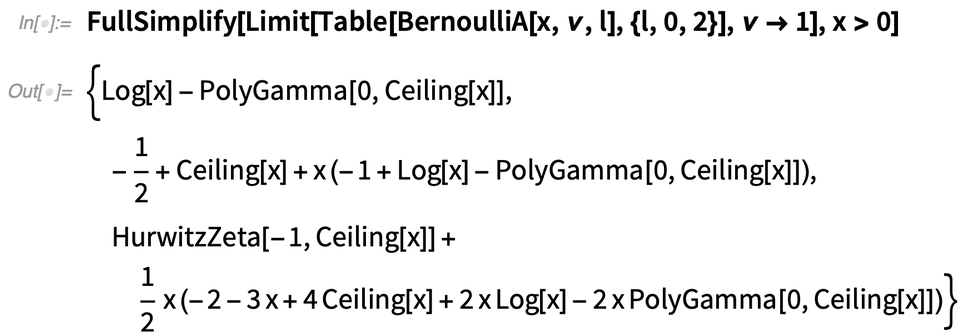✕ ```FullSimplify[ Limit[Table[BernoulliA[x, \[Nu], l], {l, 0, 2}], \[Nu] -> 1], x > 0]```

The singular Euler–Maclaurin (SEM) expansion reads: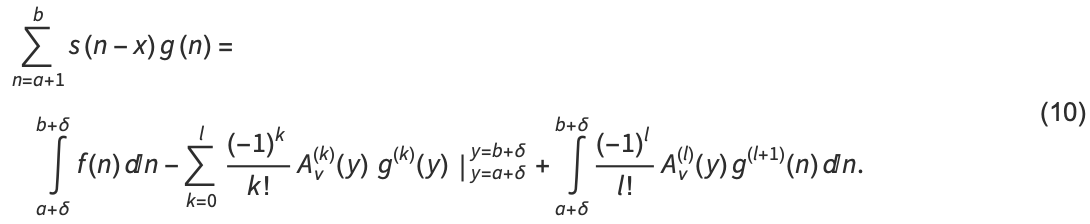If the function g is sufficiently band limited, then the error of this expansionif we discard the remainder integral—falls off exponentially in the expansion order. We have hence managed to create a new version of the Euler–Maclaurin expansion that can be applied to functions with singularities.

Let’s look at a first example.

## First Steps to Application: The Euler–Mascheroni Constant

An easy first application of the singular Euler–Maclaurin expansion is the computation of the famous Euler–Mascheroni constant γ, which is implemented in Mathematica as EulerGamma. Little is known about this elusive quantity; it is, for instance, an open problem in mathematics whether γ is an irrational number: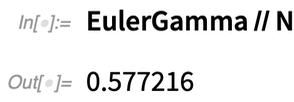✕ `EulerGamma // N`

Its value is found as a limit of the difference between a sum and an integral: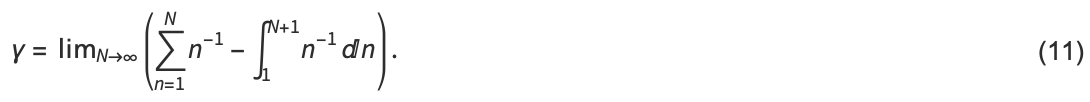We can then apply the SEM expansion to the difference between sum and integral, which consists of the zero-order contribution only:The evaluation at y = N + 1 vanishes in the limit N → ∞ and the value at y = 1 yields the desired result: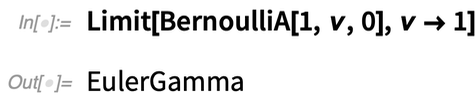✕ `Limit[BernoulliA[1, \[Nu], 0], \[Nu] -> 1]`

## Let’s Get Serious: Long-Range Forces in a Domain Wall

In order to demonstrate the performance of the expansion, we apply it to the calculation of the forces inside a 1D crystal with long-range interactions:In the previous illustration, we see a particular density modulation in the crystal, which is called a domain wall. This domain wall of width λ displaces the particles from their equilibrium positions (dashed circles). It constitutes a boundary between regions with crystalline order, where particles have equal distances.

Why are domain walls relevant in modern science? Well, domain walls occur, among other things, in layered materials. Domain walls are defects in the crystal structure that are of great importance in the design of new materials, as their presence can modify the properties of the underlying material, e.g. its elastic or electrical properties. Our goal is now to compute the force that is exerted onto the red particle by all the surrounding particles. If the crystal consists of a large number of particles, the force computation constitutes a highly challenging numerical problem.

Before we tackle the numerical task, we first need to specify the profile of the domain wall, which describes the displacement of the particles from an equidistant grid. We shall choose a domain wall whose displacement function interpolates between 0 and 1, which corresponds to a delocalized particle hole in the chain.

The displacement function of the domain wall, centered at 0, is implemented by integrating a normalized Lorentzian with width λ, from negative Infinity to y, thus obtaining a function that interpolates between 0 (–∞) and 1 (+∞):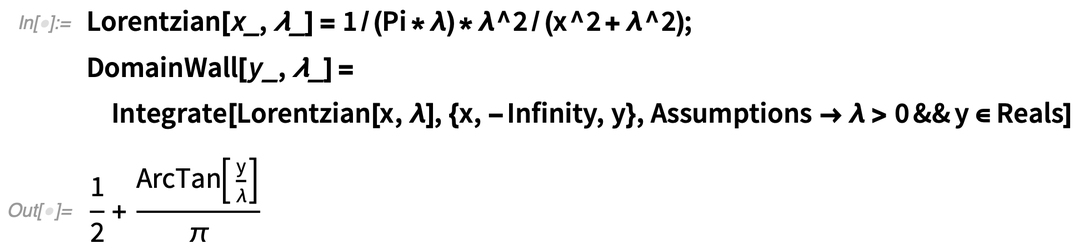✕ ```Lorentzian[x_, \[Lambda]_] = 1/(Pi*\[Lambda])*\[Lambda]^2/(x^2 + \[Lambda]^2); DomainWall[y_, \[Lambda]_] = Integrate[Lorentzian[x, \[Lambda]], {x, -Infinity, y}, Assumptions -> \[Lambda] > 0 && y \[Element] Reals]```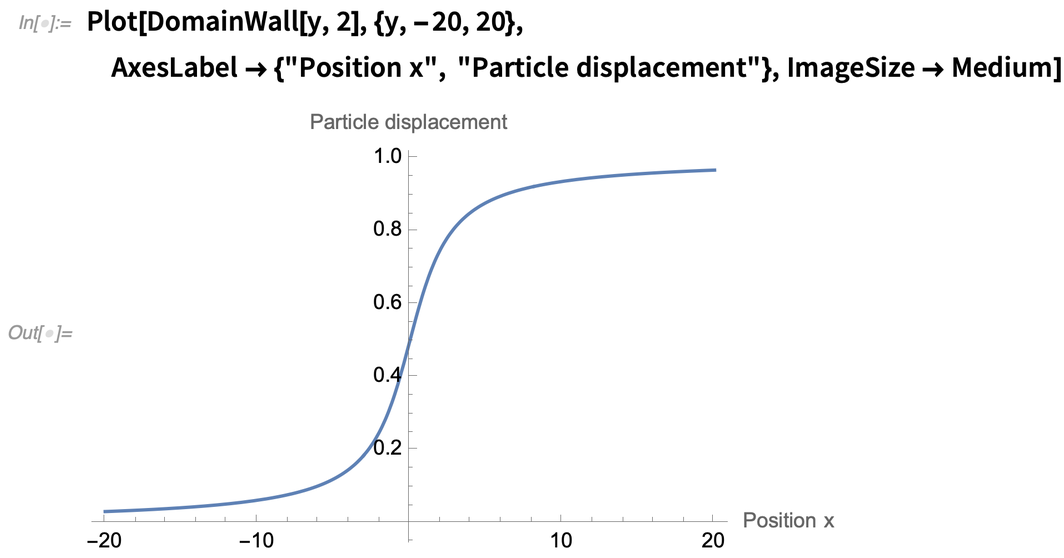✕ ```Plot[DomainWall[y, 2], {y, -20, 20}, AxesLabel -> {"Position x", "Particle displacement"}, ImageSize -> Medium]```

We then compute the force sum:After removing physical dimensions, we find thatwith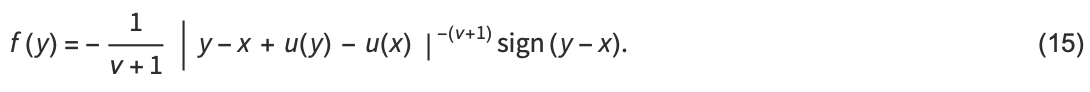Our strategy is now is to factorize the summand function into a singular factor s and smooth function factor g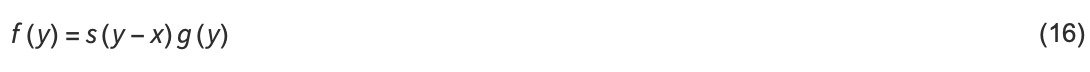with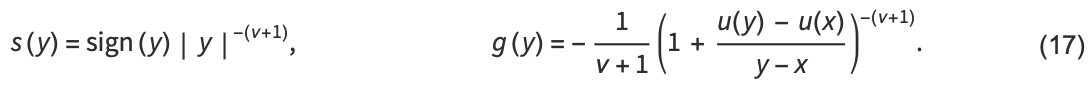We assume that the displacements are sufficiently small such that the particles remain ordered (thus the term in brackets in g is always positive and no absolute value is required). In the following, we consider the Coulomb force between charged particles (v = 1). Note that we do not apply any linearization of the forces, as our method is fully applicable to nonlinear functions g as well.

We define the functions for the interaction s, the interpolating function g and the corresponding function f. A small numerical offset is added to the value of v0 in order to simplify the implementation: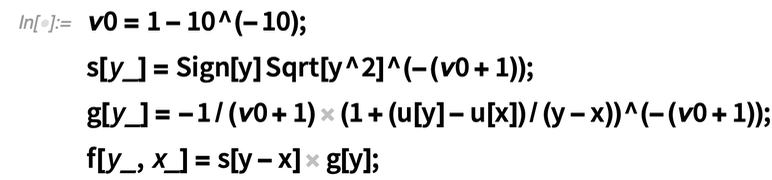✕ ```\[Nu]0 = 1 - 10^(-10); s[y_] = Sign[y] Sqrt[y^2]^(-(\[Nu]0 + 1)); g[y_] = -1/(\[Nu]0 + 1) (1 + (u[y] - u[x])/(y - x))^(-(\[Nu]0 + 1)); f[y_, x_] = s[y - x] g[y];```

We subsequently specify the size of the crystal and of the domain wall. In the first case, we opt for a microscopic crystal of 2,000 particles and a domain wall width of 10 atomic distances. In the second example, we shall explore what happens if the crystal takes a macroscopic size with 2 × 1010 particles with a domain wall of width λ = 105: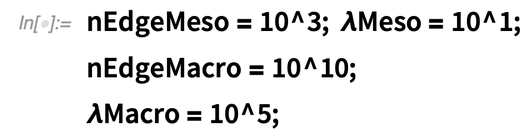✕ ```nEdgeMeso = 10^3; \[Lambda]Meso = 10^1; nEdgeMacro = 10^10; \[Lambda]Macro = 10^5;```

In the physics community, the force sum is often simply replaced by an integral. Let us now investigate how well this integral approximation reproduces the exact forces in the two cases.

We first generate tables for the respective forces.

## Crisis of the Integral Approximation and Catharsis

The following plot compares the forces obtained by exact summation to the integral approximation.

We show the exact forces (blue dots) and the forces computed using the integral approximation (orange line) for the mesoscopic chain: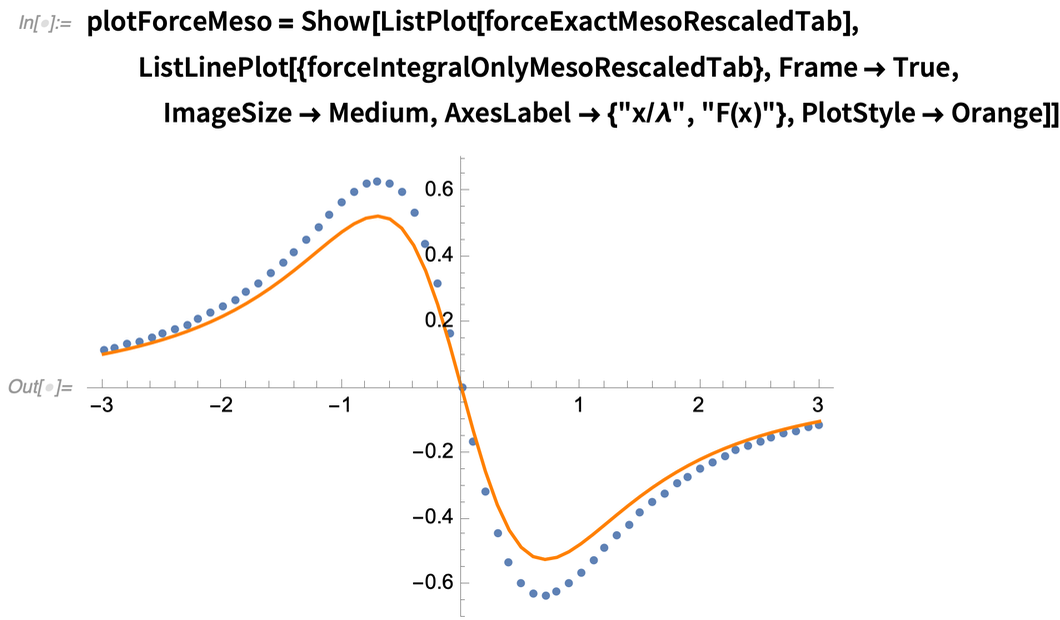✕ ```plotForceMeso = Show[ListPlot[forceExactMesoRescaledTab], ListLinePlot[{forceIntegralOnlyMesoRescaledTab}, Frame -> True, ImageSize -> Medium, AxesLabel -> {"x/\[Lambda]", "F(x)"}, PlotStyle -> Orange]]```

Both sum and integral show the qualitative behavior that the particles are drawn toward the domain wall. This makes sense, as the domain wall is a delocalized particle hole, which the other particles tend to refill. It is clear from the previous graph, however, that the integral approach strongly underestimates the absolute value of the force. It can thus only be used qualitatively and not for quantitative predictions of any kind. Small corrections can have a reasonably large effect on material properties. For precise predictions, a better approach than the integral approximation is needed. We now demonstrate that using our SEM expansion can drastically improve upon the precision of the integral.

Going from the integral approximation to the SEM is actually quite simple. We only need to implement the local SEM differential operator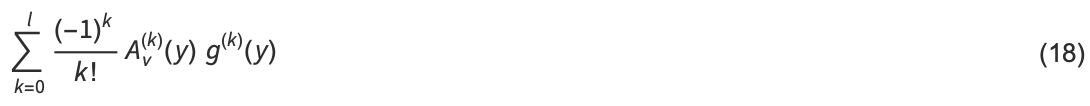and have to appropriately evaluate it at the boundaries of integration.

We define the SEM operator, using our implementation of Bernoulli-A shown previously and Mathematica’s automatic differentiation capabilities: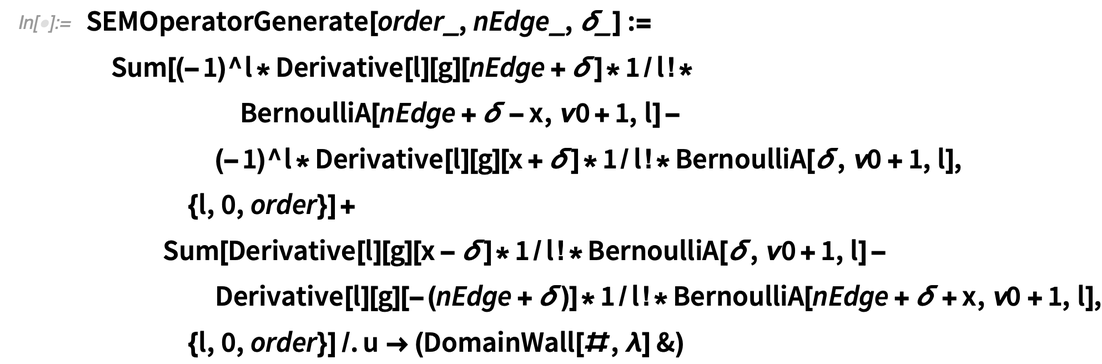✕ ```SEMOperatorGenerate[order_, nEdge_, \[Delta]_] := Sum[(-1)^l*Derivative[l][g][nEdge + \[Delta]]*1/l!* BernoulliA[nEdge + \[Delta] - x, \[Nu]0 + 1, l] - (-1)^l* Derivative[l][g][x + \[Delta]]*1/l!* BernoulliA[\[Delta], \[Nu]0 + 1, l], {l, 0, order}] + Sum[Derivative[l][g][x - \[Delta]]*1/l!* BernoulliA[\[Delta], \[Nu]0 + 1, l] - Derivative[l][g][-(nEdge + \[Delta])]*1/l!* BernoulliA[nEdge + \[Delta] + x, \[Nu]0 + 1, l], {l, 0, order}] /. u -> (DomainWall[#, \[Lambda]] &)```

We use the first-order SEM expansion with the integral offset δ0 = 1.

The expansion parameters are set and the SEM approximation to the force sum is defined:✕ ```SEMOrder = 1; \[Delta]0 = 1; ForceSEMMeso[x_, \[Lambda]_] = ForceIntegralOnly[x, \[Lambda], \[Delta]0, nEdgeMeso] - SEMOperatorGenerate[SEMOrder, nEdgeMeso, \[Delta]0];```

Once again, we compute an approximation to the forces, but now with the additional SEM correction.

We compute the SEM approximation of the force sums, using an appropriate rescaling with the domain wall width. ParallelTable allows the computation to be carried out in parallel:✕ ```forceMesoRescaledTab = ParallelTable[{x/\[Lambda]Meso, \[Lambda]Meso^2 ForceSEMMeso[ x, \[Lambda]Meso]}, {x, -3*\[Lambda]Meso, 3*\[Lambda]Meso, Floor[1 + \[Lambda]Meso/100]}];```

Finally, we compare the exact forces (blue dots) to the integral approximation (orange line) and the SEM expansion (red line):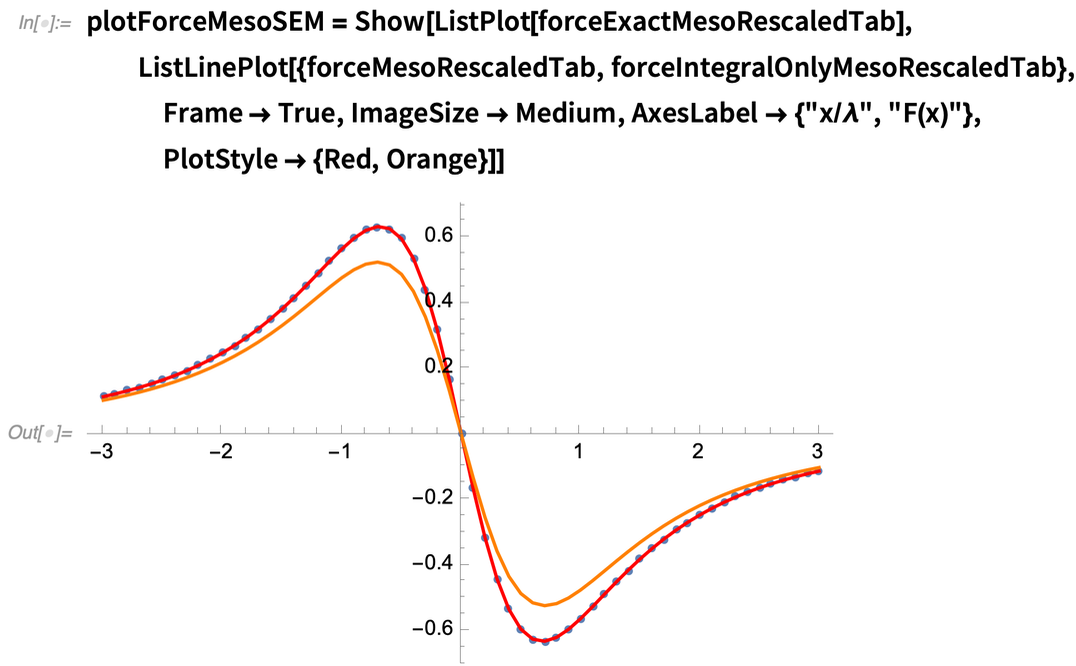✕ ```plotForceMesoSEM = Show[ListPlot[forceExactMesoRescaledTab], ListLinePlot[{forceMesoRescaledTab, forceIntegralOnlyMesoRescaledTab}, Frame -> True, ImageSize -> Medium, AxesLabel -> {"x/\[Lambda]", "F(x)"}, PlotStyle -> {Red, Orange}]]```

Using just the first-order SEM, which amounts to computing only a single derivative of g, we reach a precision that makes the SEM approximation visually indistinguishable from the exact result. Keep in mind that the correction is of local nature and can be easily computed. Therefore, we can now compute much larger particle numbers, for which the computation of the exact forces by summation is impossible.

We now move on to the macroscopic system, computing the forces in a crystal of 2 × 1010 particles and a domain wall of width λ = 105.

The forces in the macroscopic crystal computed from the SEM expansion (blue line) and from the integral approximation (orange line) are displayed: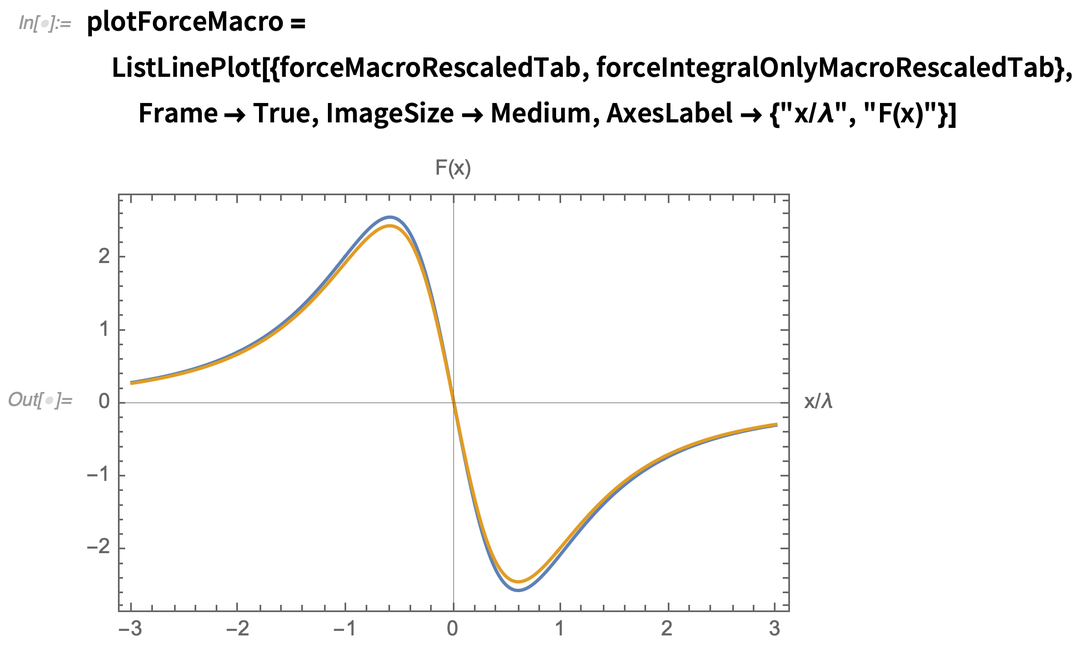✕ ```plotForceMacro = ListLinePlot[{forceMacroRescaledTab, forceIntegralOnlyMacroRescaledTab}, Frame -> True, ImageSize -> Medium, AxesLabel -> {"x/\[Lambda]", "F(x)"}]```

Here, both the integral approximation (orange) and the SEM expansion (blue) remain computable, as both exhibit a runtime that does not depend on the system size N. The evaluation of the exact sum, however, has become numerically infeasible. We see that even as N is macroscopic (at a typical inter-particle distance of 10–10m, the crystal would be two meters long), there is still a noticeable difference between the integral approximation and the SEM expansion. Here we see a very interesting effect, namely that finite-size corrections in 1D systems with Coulomb interactions remain relevant at all scales and can never really be neglected.

## Summing It Up

Centuries have passed since Euler and Maclaurin formulated the first version of the summation formula. Our modern version of the expansion now yields the promise of making an impact in modern science: resolving open problems on how to correctly deal with singularities on the way from the discrete to the continuous.

 A. A. Buchheit and T. Keßler, “Singular Euler–Maclaurin Expansion,” arXiv.org. (Apr 20, 2021) arXiv:2003.12422v3.
 A. A. Buchheit and T. Keßler, “Singular Euler–Maclaurin Expansion on Multidimensional Lattices,” arXiv.org. (Feb 22, 2021) arXiv:2102.10941.

Andreas A. Buchheit recently finished his PhD in applied mathematics in the group of Prof. Rjasanow at Saarland University, Saarbrücken, Germany, after graduating with a master’s degree in theoretical physics. His research focuses on the efficient simulation of long-range interacting systems in solid-state and quantum physics. He has been working with Mathematica for many years, using it for experimental mathematics in the search for the correct formulation of new theories.

Torsten Keßler is a PhD student in the group of Prof. Rjasanow in the mathematics department at Saarland University. Before that, he graduated with a bachelor’s degree in physics and a master’s degree in applied mathematics. His research focuses on numerical methods for kinetic equations, in particular the Boltzmann equation for rarefied gases and kinetic models for plasma dynamics.

Recently, both authors have been investigating the relation between sums and integrals with singular kernels with applications to numerical analysis and to ab-initio calculations in condensed matter physics.

 Get full access to the latest Wolfram Language functionality with a Mathematica 12.3 or Wolfram|One trial.